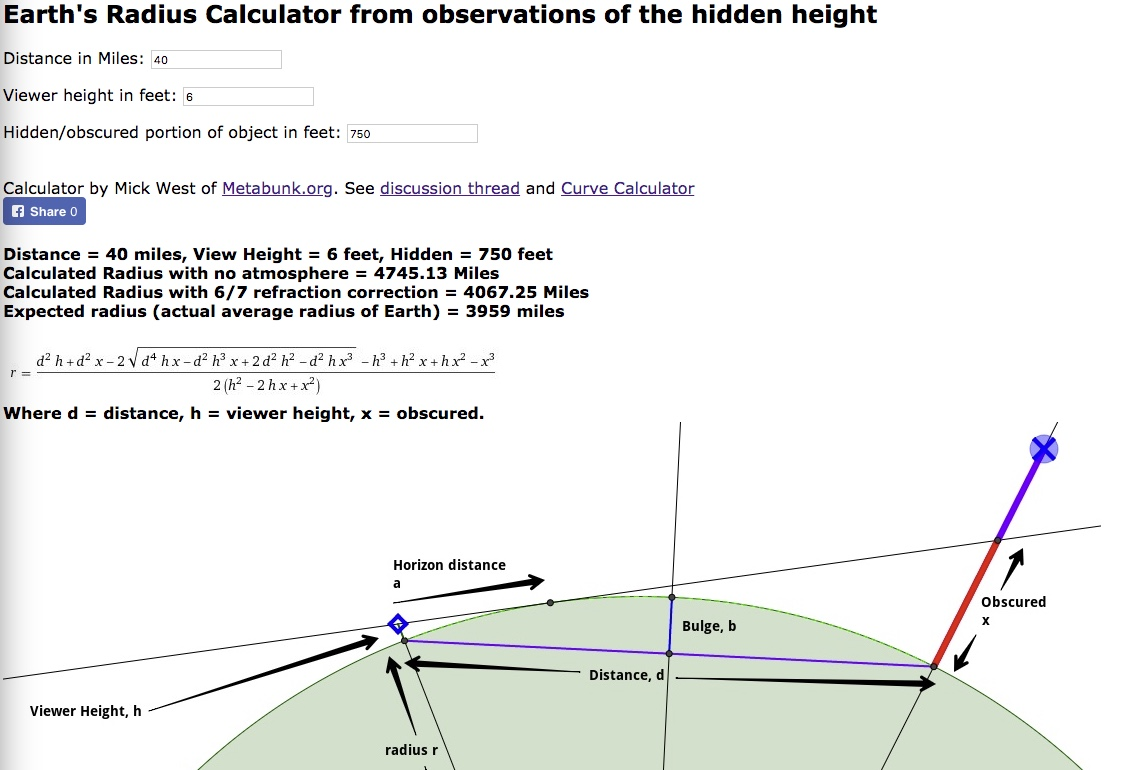# Earth's Radius Calculator (from the amount a distant object is obscured)

#### Mick West

Staff member
By simply observing how much of an object is hidden by the ocean (or lake) horizon we can calculate the radius of the Earth. Take the three values (h = height of camera about the water, d = distance to object, x = amount of object hidden) and get the radius of the earth (r)For the math we take the curve calculator and just reverse the equations. We have:

Distance to horizon, a = sqrt((r+h)*(r+h) - r*r)
Amount obscured x = sqrt(a*a - 2*a*d + d*d + r*r)-r

Substitute the first equation for a into the second to get x in terms of r, h and d
x = sqrt(((r+h)*(r+h) - r*r) - 2*sqrt(((r+h)*(r+h) - r*r))*d + d*d + r*r)-r

Solve for r:
r = (d^2*h+d^2*x +/- 2 * sqrt(d^4*h*x-d^2*h^3*x+2*d^2*h^2-d^2*h*x^3) - h^3 +h^2*x+h*x^2-x^3)/(2*(h^2-2*h*x+x^2))))

The negative solution is the correct one for short distances, so, in Javascript:
r = (d*d*h+d*d*x - 2 * Math.sqrt(d*d*d*d*h*x-d*d*h*h*h*x+2*d*d*h*h-d*d*h*x*x*x) - h*h*h +h*h*x+h*x*x-x*x*x)/(2*(h*h-2*h*x+x*x))

Last edited:

Related Articles